### DATEVALUE Function – Text to Date in Excel, VBA, G Sheets

This tutorial demonstrates how to use the Excel DATEVALUE Function in Excel to convert a date stored as text into a date serial number.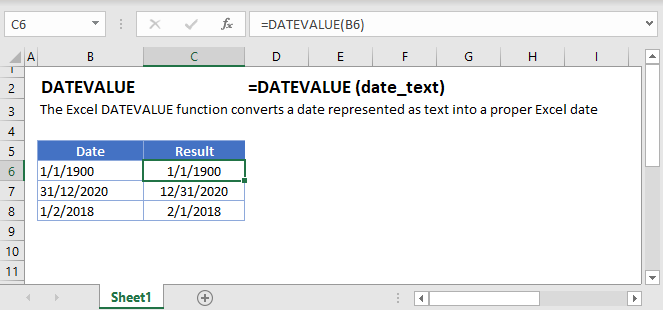## DATEVALUE Function Overview

The DATEVALUE Function Converts a date stored as text into the date’s corresponding serial number.

To use the DATEVALUE Excel Worksheet Function, select a cell and type: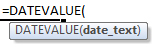## DATEVALUE Function Syntax and Inputs:

date_text – The date stored as text. Example “11/12/2015”

AutoMacro - VBA Code Generator

## DATEVALUE Examples

### Convert Date Stored as Text to Date

When working in spreadsheets it’s common to encounter dates stored as text.  You can usually identify dates stored as text because the dates are left-aligned and are proceeded by apostrophes: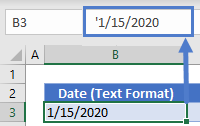These “dates” are treated as text and you’ll be unable to work with them like normal dates.

One way to convert the text to a date is with the DATEVALUE Function: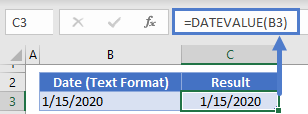Things to know about the DATEVALUE Function:

• DATEVALUE ignores time information. To convert a date+time stored as text to a number, use the VALUE Function instead. Or use the TIMEVALUE function to get the time only.
• If the year is omitted, DATEVALUE will assume the current year

## DATEVALUE in Google Sheets

The DATEVALUE Function works exactly the same in Google Sheets as in Excel: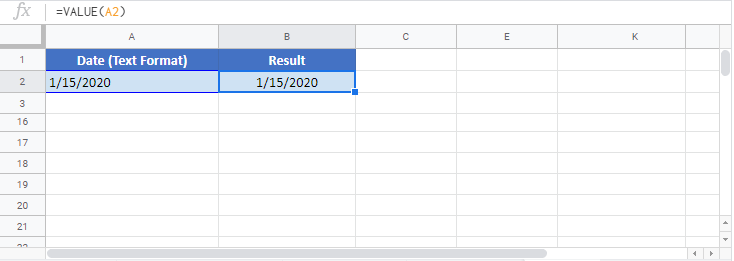## DATEVALUE Examples in VBA

You can also use the DATEVALUE function in VBA. Type:
`datevalue(date_text)`
For the function arguments (date_text, etc.), you can either enter them directly into the function, or define variables to use instead.

Lets use the DATEVALUE function from within VBA for the following  cells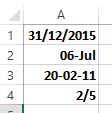Have in mind that the results returned by this function might be different on your PC, as it depends on the regional settings used by your machine.

Return to the List of all Functions in Excel

## Excel Practice Worksheet

Practice Excel functions and formulas with our 100% free practice worksheets!

• Automatically Graded Exercises
• Learn Excel, Inside Excel!